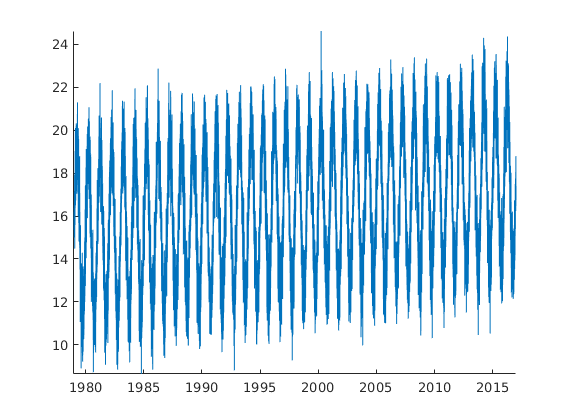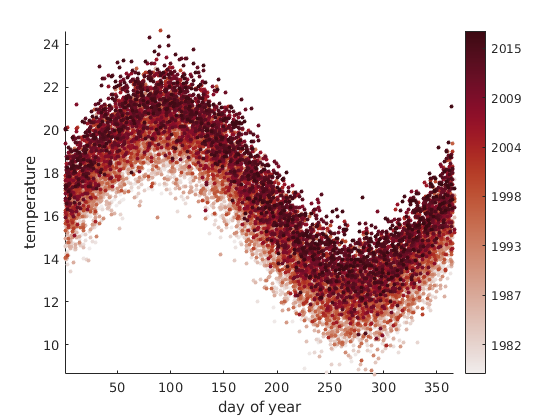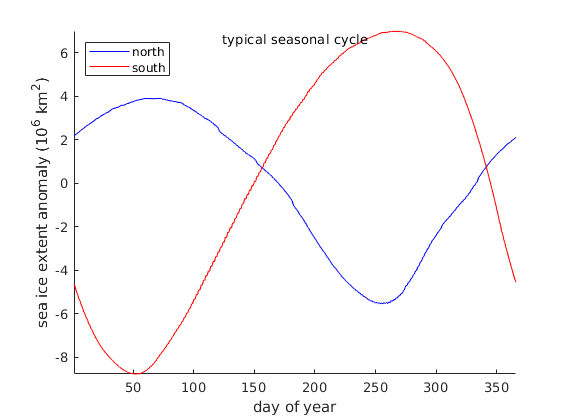# season documentation

season estimates anomalies associated with the annual cycle or of a time series.

See also: deseason, climatology, sinefit, sineval, and sinefit_bootstrap.

Back to Climate Data Tools Contents

## Syntax

```[As,ts] = season(A,t)
[As,ts] = season(...,'daily')
[As,ts] = season(...,'monthly')
[As,ts] = season(...,'detrend',DetrendOption)
[As,ts] = season(...,'dim',dimension)
As = season(...,'full')```

## Description

[As,ts] = season(A,t) gives the typical seasonal (aka annual) cycle of the time series A corresponding to times t in datenum or datetime format. If t is daily, outputs ts is 1 to 366 and As will contain average values for each of the 366 days of the year. If inputs are monthly, ts is 1:12 and As will contain average values for each of the 12 months of the year.

[As,ts] = season(...,'daily') specifies directly that inputs are daily resolution. The season function will typically figure this out automatically, but if you have large missing gaps in your data you may wish to ensure correct results by specifying daily.

[As,ts] = season(...,'monthly') as above, but forces monthly solution.

[As,ts] = season(...,'detrend',DetrendOption) specifies a baseline relative to which seasonal anomalies are determined. Options are 'linear', 'quadratic', or 'none'. By default, anomalies are calculated after removing the linear least squares trend, but if, for example, warming is strongly nonlinear, you may prefer the 'quadratic' option. Default is 'linear'.

[As,ts] = season(...,'dim',dimension) specifies a dimension along which to assess seasons. By default, if A is 1D, seasonal cycle is returned along the nonsingleton dimension; if A is 2D, season is performed along dimension 1 (time marches down the rows); if A is 3D, season is performed along dimension 3.

As = season(...,'full') returns As for the entire time series A. This is a convenient option for looking at the components of a long time series separately.

## Example 1: A single time series

Consider this time series. Let's call it temperature:

```t = datenum('jan 1, 1979'):datenum('dec 31, 2016');

% Sinusoidal seasonal signal + warming trend + noise + some mean value:
T = 4*sin(doy(t,'decimalyear')*2*pi) + (t-min(t))/5e3 + randn(size(t)) + 15;

plot(t,T)
axis tight
box off
datetick('x','keeplimits')
```You can see the temperature has a seasonal cycle, but there's a gradual warming over time and there's also some random noise in there. To see the structure of the seasonal cycle a little better, we can look at the same time series as a function of day of year using the doy function. We'll also use the cbdate function to format the colorbar dates.

```figure
scatter(doy(t),T,10,t,'filled')
axis tight

colorbar
cbdate('yyyy')
xlabel 'day of year'
ylabel 'temperature'
cmocean amp
```And again you can see the temperature rising over time, but by plotting as a function of doy we get to see the structure of the seasonal cycle a little better. Now what if we want to separate the effects of long-term warming from the seasonal cycle?

```Ts = season(T,t);

figure
plot(1:366,Ts)
box off
axis tight
xlabel 'day of year'
ylabel 'seasonal temperature cycle'
```Above we see the seasonal cycle that has an amplitude of 4 degrees, just as we defined it. Some noise remains because each day of the year represents the average of 38 noisy measurements. Nonetheless, it's our best approximation of the seasonal cycle, and it's pretty close.

## Example 2: Multiple time series at once

Let's say you have several rows of data, each containing a different time series. The data might look like this (we're building on the time series T from above)

```T2 = [T + (t-min(t))/2e3;
T - 15*cos(doy(t,'decimalyear')*2*pi) + (t-min(t))/1e2;
T + ((t-min(t))/2.5e3).^3.1];

figure
plot(t,T2)
axis tight
box off
datetick('x','keeplimits')
legend('first row of T2','second row of T2','third row of T2','location','northwest')
legend boxoff
```What's the annual cycle like for each of those time series? Use season, but specify dimension 2 because each row of T2 contains a time series. If each column of T2 contained a time series, the default dimension 1 would be what we want.

Let's also get the whole time series with the 'full' option rather than the default days 1 to 366.

```T2_full = season(T2,t,'dimension',2,'full');

figure
plot(t,T2_full)
axis([datenum('jan 1, 1995') datenum('jan 1, 2010') -16 16])
box off
datetick('x','keeplimits')

legend('first row of T2','second row of T2','third row of T2','location','northwest')
```Above we see that season has extracted the seasonal cycles of each time series in T2. Even row 3 of T2, which has a long term trend that scales to the power of 3.1 is sufficiently analyzed using the default linear detrending. However, if linear detrending is insufficient for your application, use the 'detrend','quadratic' option to step it up a notch.

## Removing seasonal cycles

For convenience, the Climate Data Toolbox has a deseason function, but it's worth noting here, that the deseason function removes seasonal cycles just by subtracting the seasonal component from the original signals, like this:

```T_deseasoned = T2 - T2_full;

figure
plot(t,T2)
hold on

plot(t,T_deseasoned)
axis tight
box off
datetick('x','keeplimits')
legend('first row of T2','second row of T2','third row of T2','location','northwest')
legend boxoff
```## Example 3: Real Sea Ice Data

The examples above used some artifical data we created. But how does this function apply to real data? Let's compare the northern and southern hemisphere seasonal cycles of sea ice extent. Here's what the full time series look like:

```load seaice_extent

figure
plot(t,extent_N,'b')
hold on
plot(t,extent_S,'r')

axis tight
box off
legend('north','south','location','northwest')
```So what's a typical year of sea ice look like?

```figure
plot(season(extent_N,t),'b')
hold on
plot(season(extent_S,t),'r')

axis tight
box off
xlabel 'day of year'
ylabel('sea ice extent anomaly (10^6 km^2)')
ntitle(' typical seasonal cycle ')
legend('north','south','location','northwest')
```## Example 4: Gridded reanalysis data

Let's explore the typical seasonal cycles of sea surface temperatures. Here's a monthly dataset of dimensions 60x55x802:

```load pacific_sst

sst_season = season(sst,t);
```

That gives us sst_season, which is 60x55x12, representing one 60x55 grid for each month of the year. Take the difference between the typical maximum and minimum temperatures to get a sense of how strong the seasons are in different parts of the Pacific:

```T_range = max(sst_season,[],3)-min(sst_season,[],3);

figure
imagescn(lon,lat,T_range)
cb = colorbar;
ylabel(cb,'magnitude of seasonal cycle (\circ C)')
cmocean amp
```That's not surprising--Seasonality is strongest close to the poles and in shallow waters.

## How this function works

By default, the season function removes the linear trend from a time series, then determines the climatology of the time series by averaging all detrended data for each day (or month) of the year.

For daily data, Leap Years present a challenge. This function calculates the average temperature for February 28th by averaging all data corresponding to the 59th day of the year. But what to do about March 1st? Most years March 1st is the 60th day of the year, but every fourth year March 1st is the 61st day of the year.

This function converts all dates to the day of the year, and calculates seasonal cycles as a function of day of the year. Since there are far fewer years with 366 days, applying the same practice of averaging all data from day 366 would make day 366 sensitive to outliers. Thus, for day 366, the season function averages all data from day 365, day 366, and day 1 of all years.

## Seasons vs Climatology

CDT has a function called season and another function called climatology. The only difference is that the output of climatology includes the mean of the variable, whereas the output of season will always have a 0 mean value. Accordingly, the deseason function removes the seasonal component of variability while preserving the overall mean and trends.

In general, CDT assumes that a multi-year record of a variable sampled at subannual resolution can described by

`y = y_0 + y_tr + y_season + y_var + y_noise`

where

• y_0 is the long-term mean,
• y_trend is the long-term trend,
• y_season is the typical seasonal anomaly, which the season function obtains after detrending and removing the mean,
• y_var represents interannual variability, and
• y_noise is everything else

In this model,

`y_climatology = y_0 + y_season`

## Other ways to define seasonality

For yet another way to define seasonality, see sinefit, sineval, and sinefit_bootstrap.

## Author Info

This function was written by Chad A. Greene of the University of Texas Institute for Geophysics (UTIG), July 2017.